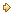Visitors Online: 77 | Friday 24th May 2019

CBSE Guess > Papers > Question Papers > Class XII > 2004 > Economics > Outside Delhi Set-II

ECONOMICS (Set II—Outside Delhi)

Except for the following questions, all the remaining questions have been asked in Set I.

SECTION - A

Q. 1. Answer the following, questions: 1x4
(i) State the law of supply.
(ii) In which market form are average revenue and marginal revenue of a firm always equal?
(iii) Define total revenue.
(iv) What is meant by price elasticity of demand?

Q. 4. Give three reasons for a righward shift of supply curve of a commodity. 3

Q. 7. Complete the following table: 4

 Output (units) Total Revenue (Rs.) Marginal Revenue (RS.) Averge Revenue (Rs.) 1 2 3 4 14 24 24 16 — — — — — — — —

Q. 9. When the price of a commodity falls from Rs. 10 per unit to Re. 9 per unit, its quantity supplied falls by 20 per cent. Calculate its price elasticity of supply. 4

SECTION - B

Q. 13. Answer the following questions: 1x4
(i) Give an example of a micro-economic study.
(ii) What is meant by revenue deficit?
(iii) What is macro-economics?
(iv) When will there be a surplus in balance of trade?

Q. 15. Calculate Net National Disposable Income from the following data: 3

 Rs. (Crores) (i) Gross domestic product at market price (ii) Net factor income form abroad (iii) Net indirect taxes (iv) Consumption of fixed capital (v) Net current transfers from rest of the world. 1,000 (-) 20 120 100 50

Q. 16. In an economy, the marginal propensity to consume is 0.95. Investment is increased by Rs. 100 crores. Calculate the total increase in income and consumption expenditures. 3

Q. 17. What is the basis of classifying government receipts into revenue receipts and capital receipts? Give an example of each. 3

Q. 23. From the following data calculate National Income by (i) income method and (ii) expenditure method:3, 3

 Rs. (Crores) (i) Compensation of employees (ii) Government final consumption expenditure (iii) Net factor income from abroad (iv) Net exports (v) Profits (vi) Net indirect tax (vii) Mixed income of self-employed (viii) Rent (ix) Interest (x) Private final consumption expenditure (xi) Net domestic capital formation (xii) Consumption of fixed capital. 600 550 (-) 10 (-) 15 400 60 350 200 310 1,000 385 65
 Economics 2004 Question Papers Class XII Delhi Outside Delhi Compartment Delhi Compartment Outside DelhiSet ISet ISet ISet ISet IISet IISet IISet IISet IIISet III

CBSE 2004 Question Papers Class XII